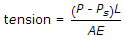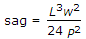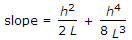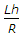# Civil Engineering - Advanced Surveying

### Exercise :: Advanced Surveying - Section 1

36.

The angle between the axis of earth and the vertical at the station of observation is called

 A. astronomical latitude B. astronomical co-latitude C. co-declination of star D. declination of star.

Explanation:

No answer description available for this question. Let us discuss.

37.

For any star to be a circumpolar star, its

 A. declination must be 0° B. declination must be 90° C. distance from the pole must be less than the latitude of the observer D. hour angle must be 180°.

Explanation:

No answer description available for this question. Let us discuss.

38.

The elevation of the star at elongation is obtained by

 A. sin α = sin φ cosec δ B. sin α = sin φ sec δ C. sin α = cos φ sec δ D. sin α = cos φ cosec δ.

Explanation:

No answer description available for this question. Let us discuss.

39.

The necessary geometrical condition for triangulation adjustment, is :

 A. The sum of the angles around a station should be 360° B. The sum of the three angles of a plane triangle should be 180° C. The sum of the eight angles of a braced quadrilateral should be 360° D. All the above.

Explanation:

No answer description available for this question. Let us discuss.

40.

The correction applied to the measured base of length L is

 A.B.where w is the weight of tape/m C.where h is height difference of end supports D. Reduction to mean sea level =E. all the above.